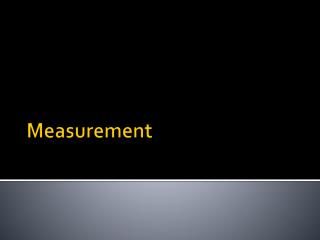DownloadDownload PresentationMeasurement

# Measurement

Download Presentation## Measurement

- - - - - - - - - - - - - - - - - - - - - - - - - - - E N D - - - - - - - - - - - - - - - - - - - - - - - - - - -
##### Presentation Transcript

1. Measurement

2. Measurement • There are two types of measurement • Quantitative measurements have a definite form and are usually expressed as numbers with units. • E.g. 3.05 grams, 12 liters, 4.5 grams/ml • Qualitative measurements are descriptive and have no numbers involved. • E.g. It gave off a “reddish gas”, It had small bumps

3. Measurement • Measurements can be either direct or derived. • Direct measurement can be taken straight from an object. • E.g. The length of an object in meters • Derived measurements must have a mathematical expression performed using several direct measurements • E.g. The volume of an object is length x width x height

4. Measurement • Density is a very important derived measurement. • Density = Mass Volume Density is an example of an intensive property because it does not depend on how much of the object you have on hand.

5. Measurement • How do you know if your measurement are true or not? • Accurate measurements are measurements that are very close to the true value of a measurement. • Precise measurements are measurements that are consistent, but not necessarily close to the true value. • The goal is to have measurements that are both accurate and precise.

6. Measurement • Think of a dart board. http://practicalanalyst.com/wp-content/uploads/2007/12/accuracy.jpg

7. Measurement • The reliability of a measurement is expressed by the number of significant figures that are present.